# Partially ordered set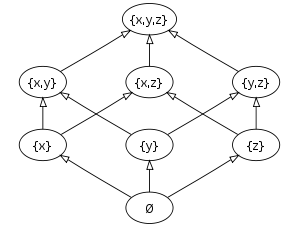The Hasse diagram of the set of all subsets of a three-element set {x, y, z}, ordered by inclusion. Sets on the same horizontal level don't share a precedence relationship. Some other pairs, such as {x} and {y,z}, do not either.

In mathematics, especially order theory, a partially ordered set (or poset) formalizes and generalizes the intuitive concept of an ordering, sequencing, or arrangement of the elements of a set. A poset consists of a set together with a binary relation that indicates that, for certain pairs of elements in the set, one of the elements precedes the other. Such a relation is called a partial order to reflect the fact that not every pair of elements need be related: for some pairs, it may be that neither element precedes the other in the poset. Thus, partial orders generalize the more familiar total orders, in which every pair is related. A finite poset can be visualized through its Hasse diagram, which depicts the ordering relation.

A familiar real-life example of a partially ordered set is a collection of people ordered by genealogical descendancy. Some pairs of people bear the descendant-ancestor relationship, but other pairs bear no such relationship.

## Formal definition

A (non-strict) partial order is a binary relation ≤ over a set P which is reflexive, antisymmetric, and transitive, i.e., which satisfies for all a, b, and c in P:

• aa (reflexivity: every element is related to itself).
• if ab and ba, then a = b (antisymmetry: there exists at most one relation between two distinct elements)
• if ab and bc, then ac (transitivity: if a first element is related to a second element, and, in turn, that element is related to a third element, then the first element is related to the third element).

In other words, a partial order is an antisymmetric preorder.

A set with a partial order is called a partially ordered set (also called a poset). The term ordered set is sometimes also used, as long as it is clear from the context that no other kind of order is meant. In particular, totally ordered sets can also be referred to as "ordered sets", especially in areas where these structures are more common than posets.

For a, b, elements of a partially ordered set P, if ab or ba, then a and b are comparable. Otherwise they are incomparable. In the figure on top-right, e.g. {x} and {x,y,z} are comparable, while {x} and {y} are not. A partial order under which every pair of elements is comparable is called a total order or linear order; a totally ordered set is also called a chain (e.g., the natural numbers with their standard order). A subset of a poset in which no two distinct elements are comparable is called an antichain (e.g. the set of singletons {{x}, {y}, {z}} in the top-right figure). An element a is said to be covered by another element b, written a<:b, if a is strictly less than b and no third element c fits between them; formally: if both ab and ab are true, and acb is false for each c with acb. A more concise definition will be given below using the strict order corresponding to "≤". For example, {x} is covered by {x,z} in the top-right figure, but not by {x,y,z}.

## Examples

Standard examples of posets arising in mathematics include:

## Extrema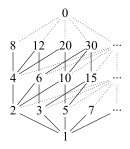Nonnegative integers, ordered by divisibility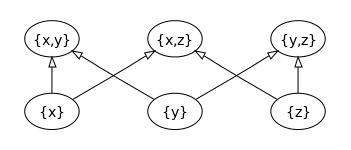The figure above with the greatest and least elements removed. In this reduced poset, the top row of elements are all maximal elements, and the bottom row are all minimal elements, but there is no greatest and no least element. The set {x, y} is an upper bound for the collection of elements {{x}, {y}}.

There are several notions of "greatest" and "least" element in a poset P, notably:

• Greatest element and least element: An element g in P is a greatest element if for every element a in P, a  g. An element m in P is a least element if for every element a in P, a  m. A poset can only have one greatest or least element.
• Maximal elements and minimal elements: An element g in P is a maximal element if there is no element a in P such that a > g. Similarly, an element m in P is a minimal element if there is no element a in P such that a < m. If a poset has a greatest element, it must be the unique maximal element, but otherwise there can be more than one maximal element, and similarly for least elements and minimal elements.
• Upper and lower bounds: For a subset A of P, an element x in P is an upper bound of A if a  x, for each element a in A. In particular, x need not be in A to be an upper bound of A. Similarly, an element x in P is a lower bound of A if a  x, for each element a in A. A greatest element of P is an upper bound of P itself, and a least element is a lower bound of P.

For example, consider the positive integers, ordered by divisibility: 1 is a least element, as it divides all other elements; on the other hand this poset does not have a greatest element (although if one would include 0 in the poset, which is a multiple of any integer, that would be a greatest element; see figure). This partially ordered set does not even have any maximal elements, since any g divides for instance 2g, which is distinct from it, so g is not maximal. If the number 1 is excluded, while keeping divisibility as ordering on the elements greater than 1, then the resulting poset does not have a least element, but any prime number is a minimal element for it. In this poset, 60 is an upper bound (though not a least upper bound) of the subset {2,3,5,10}, which does not have any lower bound (since 1 is not in the poset); on the other hand 2 is a lower bound of the subset of powers of 2, which does not have any upper bound.

## Orders on the Cartesian product of partially ordered sets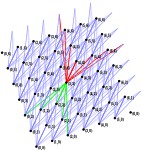Reflexive closure of strict direct product order on ℕ×ℕ. Elements covered by (3,3) and covering (3,3) are highlighted in green and red, respectively.Product order on ℕ×ℕLexicographic order on ℕ×ℕ

In order of increasing strength, i.e., decreasing sets of pairs, three of the possible partial orders on the Cartesian product of two partially ordered sets are (see figures):

All three can similarly be defined for the Cartesian product of more than two sets.

Applied to ordered vector spaces over the same field, the result is in each case also an ordered vector space.

## Sums of partially ordered sets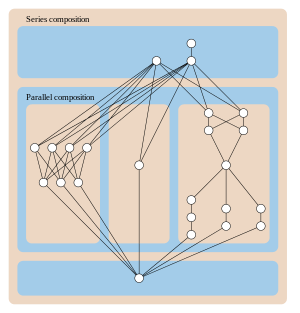Hasse diagram of a series-parallel partial order, formed as the ordinal sum of three smaller partial orders.

Another way to combine two posets is the ordinal sum (or linear sum), Z = XY, defined on the union of the underlying sets X and Y by the order aZ b if and only if:

• a, bX with aX b, or
• a, bY with aY b, or
• aX and bY.

If two posets are well-ordered, then so is their ordinal sum. The ordinal sum operation is one of two operations used to form series-parallel partial orders, and in this context is called series composition. The other operation used to form these orders, the disjoint union of two partially ordered sets (with no order relation between elements of one set and elements of the other set) is called in this context parallel composition.

## Strict and non-strict partial orders

In some contexts, the partial order defined above is called a non-strict (or reflexive, or weak) partial order. In these contexts, a strict (or irreflexive) partial order "<" is a binary relation that is irreflexive, transitive and asymmetric, i.e. which satisfies for all a, b, and c in P:

• not a < a (irreflexivity),
• if a < b and b < c then a < c (transitivity), and
• if a < b then not b < a (asymmetry; implied by irreflexivity and transitivity).

There is a 1-to-1 correspondence between all non-strict and strict partial orders.

If "≤" is a non-strict partial order, then the corresponding strict partial order "<" is the irreflexive kernel given by:

a < b if ab and ab

Conversely, if "<" is a strict partial order, then the corresponding non-strict partial order "≤" is the reflexive closure given by:

ab if a < b or a = b.

This is the reason for using the notation "≤".

Using the strict order "<", the relation "a is covered by b" can be equivalently rephrased as "a<b, but not a<c<b for any c". Strict partial orders are useful because they correspond more directly to directed acyclic graphs (dags): every strict partial order is a dag, and the transitive closure of a dag is both a strict partial order and also a dag itself.

## Inverse and order dual

The inverse or converse ≥ of a partial order relation ≤ satisfies xy if and only if yx. The inverse of a partial order relation is reflexive, transitive, and antisymmetric, and hence itself a partial order relation. The order dual of a partially ordered set is the same set with the partial order relation replaced by its inverse. The irreflexive relation > is to ≥ as < is to ≤.

Any one of the four relations ≤, <, ≥, and > on a given set uniquely determines the other three.

In general two elements x and y of a partial order may stand in any of four mutually exclusive relationships to each other: either x < y, or x = y, or x > y, or x and y are incomparable (none of the other three). A totally ordered set is one that rules out this fourth possibility: all pairs of elements are comparable and we then say that trichotomy holds. The natural numbers, the integers, the rationals, and the reals are all totally ordered by their algebraic (signed) magnitude whereas the complex numbers are not. This is not to say that the complex numbers cannot be totally ordered; we could for example order them lexicographically via x+iy < u+iv if and only if x < u or (x = u and y < v), but this is not ordering by magnitude in any reasonable sense as it makes 1 greater than 100i. Ordering them by absolute magnitude yields a preorder in which all pairs are comparable, but this is not a partial order since 1 and i have the same absolute magnitude but are not equal, violating antisymmetry.

## Mappings between partially ordered sets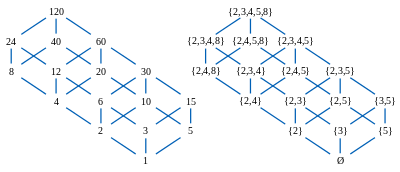Order isomorphism between the divisors of 120 (partially ordered by divisibility) and the divisor-closed subsets of {2,3,4,5,8} (partially ordered by set inclusion)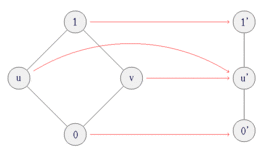Order-preserving, but not order-reflecting (since f(u)≤f(v), but not u≤v) map.

Given two partially ordered sets (S,≤) and (T,≤), a function f: ST is called order-preserving, or monotone, or isotone, if for all x and y in S, xy implies f(x) ≤ f(y). If (U,≤) is also a partially ordered set, and both f: ST and g: TU are order-preserving, their composition (gf): SU is order-preserving, too. A function f: ST is called order-reflecting if for all x and y in S, f(x) ≤ f(y) implies xy. If f is both order-preserving and order-reflecting, then it is called an order-embedding of (S,≤) into (T,≤). In the latter case, f is necessarily injective, since f(x) = f(y) implies xy and yx. If an order-embedding between two posets S and T exists, one says that S can be embedded into T. If an order-embedding f: ST is bijective, it is called an order isomorphism, and the partial orders (S,≤) and (T,≤) are said to be isomorphic. Isomorphic orders have structurally similar Hasse diagrams (cf. right picture). It can be shown that if order-preserving maps f: ST and g: TS exist such that gf and fg yields the identity function on S and T, respectively, then S and T are order-isomorphic. 

For example, a mapping f: ℕ → ℙ(ℕ) from the set of natural numbers (ordered by divisibility) to the power set of natural numbers (ordered by set inclusion) can be defined by taking each number to the set of its prime divisors. It is order-preserving: if x divides y, then each prime divisor of x is also a prime divisor of y. However, it is neither injective (since it maps both 12 and 6 to {2,3}) nor order-reflecting (since besides 12 doesn't divide 6). Taking instead each number to the set of its prime power divisors defines a map g: ℕ → ℙ(ℕ) that is order-preserving, order-reflecting, and hence an order-embedding. It is not an order-isomorphism (since it e.g. doesn't map any number to the set {4}), but it can be made one by restricting its codomain to g(ℕ). The right picture shows a subset of ℕ and its isomorphic image under g. The construction of such an order-isomorphism into a power set can be generalized to a wide class of partial orders, called distributive lattices, see "Birkhoff's representation theorem".

## Number of partial orders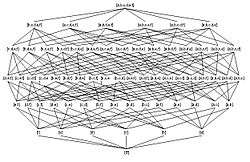Partially ordered set of set of all subsets of a six-element set {a, b, c, d, e, f}, ordered by the subset relation.

Sequence A001035 in OEIS gives the number of partial orders on a set of n labeled elements:

Number of n-element binary relations of different types
nalltransitivereflexivepreorderpartial ordertotal preordertotal orderequivalence relation
011111111
122111111
21613443322
35121716429191365
46553639944096355219752415
n2n2 2n2-n Σn
k=0

k! S(n,k)
n!Σn
k=0

S(n,k)
OEIS A002416 A006905 A053763 A000798 A001035 A000670 A000142 A000110

The number of strict partial orders is the same as that of partial orders.

If we count only up to isomorphism, we get 1, 1, 2, 5, 16, 63, 318, … (sequence A000112 in the OEIS).

## Linear extension

A partial order ≤* on a set X is an extension of another partial order ≤ on X provided that for all elements x and y of X, whenever xy, it is also the case that x * y. A linear extension is an extension that is also a linear (i.e., total) order. Every partial order can be extended to a total order (order-extension principle).

In computer science, algorithms for finding linear extensions of partial orders (represented as the reachability orders of directed acyclic graphs) are called topological sorting.

## In category theory

Every poset (and every preorder) may be considered as a category in which every hom-set has at most one element. More explicitly, let hom(x, y) = {(x, y)} if xy (and otherwise the empty set) and (y, z)∘(x, y) = (x, z). Such categories are sometimes called posetal.

Posets are equivalent to one another if and only if they are isomorphic. In a poset, the smallest element, if it exists, is an initial object, and the largest element, if it exists, is a terminal object. Also, every preordered set is equivalent to a poset. Finally, every subcategory of a poset is isomorphism-closed.

## Partial orders in topological spaces

If P is a partially ordered set that has also been given the structure of a topological space, then it is customary to assume that {(a, b) : a b} is a closed subset of the topological product space. Under this assumption partial order relations are well behaved at limits in the sense that if,and ai  bi for all i, then a  b.

## Interval

For ab, the closed interval [a,b] is the set of elements x satisfying axb (i.e. ax and xb). It contains at least the elements a and b.

Using the corresponding strict relation "<", the open interval (a,b) is the set of elements x satisfying a < x < b (i.e. a < x and x < b). An open interval may be empty even if a < b. For example, the open interval (1,2) on the integers is empty since there are no integers i such that 1 < i < 2.

Sometimes the definitions are extended to allow a > b, in which case the interval is empty.

The half-open intervals [a,b) and (a,b] are defined similarly.

A poset is locally finite if every interval is finite. For example, the integers are locally finite under their natural ordering. The lexicographical order on the cartesian product ℕ×ℕ is not locally finite, since e.g. (1,2)≤(1,3)≤(1,4)≤(1,5)≤...≤(2,1). Using the interval notation, the property "a is covered by b" can be rephrased equivalently as [a,b] = {a,b}.

This concept of an interval in a partial order should not be confused with the particular class of partial orders known as the interval orders.

1. Merrifield, Richard E.; Simmons, Howard E. (1989). Topological Methods in Chemistry. New York: John Wiley & Sons. p. 28. ISBN 0-471-83817-9. Retrieved 27 July 2012. A partially ordered set is conveniently represented by a Hasse diagram...
2. Simovici, Dan A. & Djeraba, Chabane (2008). "Partially Ordered Sets". Mathematical Tools for Data Mining: Set Theory, Partial Orders, Combinatorics. Springer. ISBN 9781848002012.
3. Neggers, J.; Kim, Hee Sik (1998), "4.2 Product Order and Lexicographic Order", Basic Posets, World Scientific, pp. 62–63, ISBN 9789810235895
4. Davey, B. A.; Priestley, H. A., Introduction to Lattices and Order (Second Edition), 2002, p. 17-18
5. P. R. Halmos (1974). Naive Set Theory. Springer. p. 82. ISBN 978-1-4757-1645-0.
6. Flaška, V.; Ježek, J.; Kepka, T.; Kortelainen, J. (2007). Transitive Closures of Binary Relations I (PDF). Prague: School of Mathematics - Physics Charles University. p. 1. Lemma 1.1 (iv). Note that this source refers to asymmetric relations as "strictly antisymmetric".
7. Davey, B. A.; Priestley, H. A. (2002). "Maps between ordered sets". Introduction to Lattices and Order (2nd ed.). New York: Cambridge University Press. pp. 23–24. ISBN 0-521-78451-4. MR 1902334.
8. Jech, Thomas (2008) . The Axiom of Choice. Dover Publications. ISBN 0-486-46624-8.
9. Ward, L. E. Jr (1954). "Partially Ordered Topological Spaces". Proceedings of the American Mathematical Society. 5 (1): 144–161. doi:10.1090/S0002-9939-1954-0063016-5
• Deshpande, Jayant V. (1968). "On Continuity of a Partial Order". Proceedings of the American Mathematical Society. 19 (2): 383–386. doi:10.1090/S0002-9939-1968-0236071-7.
• Schröder, Bernd S. W. (2003). Ordered Sets: An Introduction. Birkhäuser, Boston.
• Stanley, Richard P. Enumerative Combinatorics 1. Cambridge Studies in Advanced Mathematics. 49. Cambridge University Press. ISBN 0-521-66351-2.Wikimedia Commons has media related to Hasse diagram.
• A001035: Number of posets with n labeled elements in the OEIS
• A000112: Number of posets with n unlabeled elements in the OEIS